Units: SI(mm) & US(in).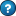Copy for Native Excel:
Purpose of calculation:
Calculate bending moments, shear, slope and deflection of a built in beam under a point load.
Calculation Reference
Roark's Formulas for Stress and Strain (7th Edition)
Table 8.1 case 1d - Left end fixed, right end fixed
Calculation Validation
Independent check on deflection checked at www.xcalcs.com
l = 5000 mm in
E = 210000 N/mm² lbf/in²
I = 1900000 mm⁴ in⁴
W = 5000 N lbf
a = 4000 mm in
Boundary Values
Vertical reaction at left end
RA = =(E14*(E11-E15)^2*(E11+2*E15))/(E11^3) N lbf
Moment reaction at left end
MA = =(-E14*E15*(E11-E15)^2)/(E11^2) Nmm lbfin
End slope at left end
Deflection at left end
yA = 0 mm in
Vertical reaction at right end
RB = =(E14*E15^2*(3*E11-2*E15))/(E11^3) N lbf
Moment reaction at right end
MB = =(-E14*E15^2*(E11-E15))/(E11^2) Nmm lbfin
End slope at right end
Deflection at right end
yB = 0 mm in
Selected maximum values of moments and deformations
Max + M = 2Wa²(l-a)²/l³ at x=a; max possible value = Wl/8 when a= l/2
Max -M=MA if a < l/2; max possible value = -0.1481Wl when a=1/3
Max y=-2W(l-a)²a³/3EI(l+2a)² at x=2al/(l+2a) when a > 1/2;
max possible value = -Wl3/192EI when x=a=1/2
Calculate values for any point along longitudinal axis of the beam
x = 3000 mm in x = 0 =AF55+\$E11/10 =AG55+\$E11/10 =AH55+\$E11/10 =AI55+\$E11/10 =AJ55+\$E11/10 =AK55+\$E11/10 =AL55+\$E11/10 =AM55+\$E11/10 =AN55+\$E11/10 =AO55+\$E11/10
Heavyside step function Heavyside step function
Hx = =IF(E55>\$E15,E55-\$E15,0) Hx = =IF(AF55>\$E15,AF55-\$E15,0) =IF(AG55>\$E15,AG55-\$E15,0) =IF(AH55>\$E15,AH55-\$E15,0) =IF(AI55>\$E15,AI55-\$E15,0) =IF(AJ55>\$E15,AJ55-\$E15,0) =IF(AK55>\$E15,AK55-\$E15,0) =IF(AL55>\$E15,AL55-\$E15,0) =IF(AM55>\$E15,AM55-\$E15,0) =IF(AN55>\$E15,AN55-\$E15,0) =IF(AO55>\$E15,AO55-\$E15,0) =IF(AP55>\$E15,AP55-\$E15,0)
Heavyside step function raised to the power 0 Heavyside step function raised to the power 0
Hx0 = =IF(E55>\$E15,1,0) Hx0 = =IF(AF55>\$E15,1,0) =IF(AG55>\$E15,1,0) =IF(AH55>\$E15,1,0) =IF(AI55>\$E15,1,0) =IF(AJ55>\$E15,1,0) =IF(AK55>\$E15,1,0) =IF(AL55>\$E15,1,0) =IF(AM55>\$E15,1,0) =IF(AN55>\$E15,1,0) =IF(AO55>\$E15,1,0) =IF(AP55>\$E15,1,0)
Transverse Shear Transverse Shear
V = =\$E21-\$E14*E59 N lbf V = =\$E21-\$E14*AF59 =\$E21-\$E14*AG59 =\$E21-\$E14*AH59 =\$E21-\$E14*AI59 =\$E21-\$E14*AJ59 =\$E21-\$E14*AK59 =\$E21-\$E14*AL59 =\$E21-\$E14*AM59 =\$E21-\$E14*AN59 =\$E21-\$E14*AO59 =\$E21-\$E14*AP59
Bending Moment Bending Moment
M = =\$E23+\$E21*E55-\$E14*E57 Nmm lbfin M = =\$E23+\$E21*AF55-\$E14*AF57 =\$E23+\$E21*AG55-\$E14*AG57 =\$E23+\$E21*AH55-\$E14*AH57 =\$E23+\$E21*AI55-\$E14*AI57 =\$E23+\$E21*AJ55-\$E14*AJ57 =\$E23+\$E21*AK55-\$E14*AK57 =\$E23+\$E21*AL55-\$E14*AL57 =\$E23+\$E21*AM55-\$E14*AM57 =\$E23+\$E21*AN55-\$E14*AN57 =\$E23+\$E21*AO55-\$E14*AO57 =\$E23+\$E21*AP55-\$E14*AP57
Slope Slope
θ = =\$E25+(\$E23*E55)/(\$E12*\$E13)+(\$E21*E55^2)/(2*\$E12*\$E13)-(\$E14*E57^2)/(2*\$E12*\$E13) rads rads θ = =\$E25+(\$E23*AF55)/(\$E12*\$E13)+(\$E21*AF55^2)/(2*\$E12*\$E13)-(\$E14*AF57^2)/(2*\$E12*\$E13) =\$E25+(\$E23*AG55)/(\$E12*\$E13)+(\$E21*AG55^2)/(2*\$E12*\$E13)-(\$E14*AG57^2)/(2*\$E12*\$E13) =\$E25+(\$E23*AH55)/(\$E12*\$E13)+(\$E21*AH55^2)/(2*\$E12*\$E13)-(\$E14*AH57^2)/(2*\$E12*\$E13) =\$E25+(\$E23*AI55)/(\$E12*\$E13)+(\$E21*AI55^2)/(2*\$E12*\$E13)-(\$E14*AI57^2)/(2*\$E12*\$E13) =\$E25+(\$E23*AJ55)/(\$E12*\$E13)+(\$E21*AJ55^2)/(2*\$E12*\$E13)-(\$E14*AJ57^2)/(2*\$E12*\$E13) =\$E25+(\$E23*AK55)/(\$E12*\$E13)+(\$E21*AK55^2)/(2*\$E12*\$E13)-(\$E14*AK57^2)/(2*\$E12*\$E13) =\$E25+(\$E23*AL55)/(\$E12*\$E13)+(\$E21*AL55^2)/(2*\$E12*\$E13)-(\$E14*AL57^2)/(2*\$E12*\$E13) =\$E25+(\$E23*AM55)/(\$E12*\$E13)+(\$E21*AM55^2)/(2*\$E12*\$E13)-(\$E14*AM57^2)/(2*\$E12*\$E13) =\$E25+(\$E23*AN55)/(\$E12*\$E13)+(\$E21*AN55^2)/(2*\$E12*\$E13)-(\$E14*AN57^2)/(2*\$E12*\$E13) =\$E25+(\$E23*AO55)/(\$E12*\$E13)+(\$E21*AO55^2)/(2*\$E12*\$E13)-(\$E14*AO57^2)/(2*\$E12*\$E13) =\$E25+(\$E23*AP55)/(\$E12*\$E13)+(\$E21*AP55^2)/(2*\$E12*\$E13)-(\$E14*AP57^2)/(2*\$E12*\$E13)
Deflection Deflection
y= =\$E27+\$E25*E55+(\$E23*E55^2)/(2*\$E12*\$E13)+(\$E21*E55^3)/(6*\$E12*\$E13)-(\$E14*E57^3)/(6*\$E12*\$E13) mm in y= =\$E27+\$E25*AF55+(\$E23*AF55^2)/(2*\$E12*\$E13)+(\$E21*AF55^3)/(6*\$E12*\$E13)-(\$E14*AF57^3)/(6*\$E12*\$E13) =\$E27+\$E25*AG55+(\$E23*AG55^2)/(2*\$E12*\$E13)+(\$E21*AG55^3)/(6*\$E12*\$E13)-(\$E14*AG57^3)/(6*\$E12*\$E13) =\$E27+\$E25*AH55+(\$E23*AH55^2)/(2*\$E12*\$E13)+(\$E21*AH55^3)/(6*\$E12*\$E13)-(\$E14*AH57^3)/(6*\$E12*\$E13) =\$E27+\$E25*AI55+(\$E23*AI55^2)/(2*\$E12*\$E13)+(\$E21*AI55^3)/(6*\$E12*\$E13)-(\$E14*AI57^3)/(6*\$E12*\$E13) =\$E27+\$E25*AJ55+(\$E23*AJ55^2)/(2*\$E12*\$E13)+(\$E21*AJ55^3)/(6*\$E12*\$E13)-(\$E14*AJ57^3)/(6*\$E12*\$E13) =\$E27+\$E25*AK55+(\$E23*AK55^2)/(2*\$E12*\$E13)+(\$E21*AK55^3)/(6*\$E12*\$E13)-(\$E14*AK57^3)/(6*\$E12*\$E13) =\$E27+\$E25*AL55+(\$E23*AL55^2)/(2*\$E12*\$E13)+(\$E21*AL55^3)/(6*\$E12*\$E13)-(\$E14*AL57^3)/(6*\$E12*\$E13) =\$E27+\$E25*AM55+(\$E23*AM55^2)/(2*\$E12*\$E13)+(\$E21*AM55^3)/(6*\$E12*\$E13)-(\$E14*AM57^3)/(6*\$E12*\$E13) =\$E27+\$E25*AN55+(\$E23*AN55^2)/(2*\$E12*\$E13)+(\$E21*AN55^3)/(6*\$E12*\$E13)-(\$E14*AN57^3)/(6*\$E12*\$E13) =\$E27+\$E25*AO55+(\$E23*AO55^2)/(2*\$E12*\$E13)+(\$E21*AO55^3)/(6*\$E12*\$E13)-(\$E14*AO57^3)/(6*\$E12*\$E13) =\$E27+\$E25*AP55+(\$E23*AP55^2)/(2*\$E12*\$E13)+(\$E21*AP55^3)/(6*\$E12*\$E13)-(\$E14*AP57^3)/(6*\$E12*\$E13)
Plot Deflection for 10 Points along the Length of the BeamCopy for XLC Enhanced Excel:
Purpose of calculation:
Calculate bending moments, shear, slope and deflection of a built in beam under a point load.
Calculation Reference
Roark's Formulas for Stress and Strain (7th Edition)
Table 8.1 case 1d - Left end fixed, right end fixed
Calculation Validation
Independent check on deflection checked at www.xcalcs.com
l = 5000 mm in
E = 210000 N/mm² lbf/in²
I = 1900000 mm⁴ in⁴
W = 5000 N lbf
a = 4000 mm in
Boundary Values
Vertical reaction at left end
RA = =OVD(E14*(E11-E15)^2*(E11+2*E15))/UND(E11^3) N lbf =EQS(E21,"Units= ; EqnPrefix=Eqn.; EqnNo= 0; Multiplication= 0; ShowWorking= 0; EqnStyle= 0; Eqp\$H\$25_0")
Moment reaction at left end
MA = =OVD(-E14*E15*(E11-E15)^2)/UND(E11^2) Nmm lbfin =EQS(E23,"Units= ; EqnPrefix=Eqn.; EqnNo= 0; Multiplication= 0; ShowWorking= 0; EqnStyle= 0; Eqp\$H\$27_0")
End slope at left end
Deflection at left end
yA = 0 mm in
Vertical reaction at right end
RB = =OVD(E14*E15^2*(3*E11-2*E15))/UND(E11^3) N lbf =EQS(E30,"Units= ; EqnPrefix=Eqn.; EqnNo= 0; Multiplication= 0; ShowWorking= 0; EqnStyle= 0; Eqp\$H\$34_0")
Moment reaction at right end
MB = =OVD(-E14*E15^2*(E11-E15))/UND(E11^2) Nmm lbfin =EQS(E32,"Units= ; EqnPrefix=Eqn.; EqnNo= 0; Multiplication= 0; ShowWorking= 0; EqnStyle= 0; Eqp\$H\$36_0")
End slope at right end
Deflection at right end
yB = 0 mm in
Selected maximum values of moments and deformations
Max + M = 2Wa²(l-a)²/l³ at x=a; max possible value = Wl/8 when a= l/2
Max -M=MA if a < l/2; max possible value = -0.1481Wl when a=1/3
Max y=-2W(l-a)²a³/3EI(l+2a)² at x=2al/(l+2a) when a > 1/2;
max possible value = -Wl3/192EI when x=a=1/2
Calculate values for any point along longitudinal axis of the beam
x = 3000 mm in x = 0 =AF55+\$E11/10 =AG55+\$E11/10 =AH55+\$E11/10 =AI55+\$E11/10 =AJ55+\$E11/10 =AK55+\$E11/10 =AL55+\$E11/10 =AM55+\$E11/10 =AN55+\$E11/10 =AO55+\$E11/10
Heavyside step function Heavyside step function
Hx = =IF(E55>\$E15,E55-\$E15,0) =EQS(E57,"Units= ; EqnPrefix=Eqn.; EqnNo= 0; Multiplication= 0; ShowWorking= 0; EqnStyle= 0; Eqp\$H\$61_0") Hx = =IF(AF55>\$E15,AF55-\$E15,0) =IF(AG55>\$E15,AG55-\$E15,0) =IF(AH55>\$E15,AH55-\$E15,0) =IF(AI55>\$E15,AI55-\$E15,0) =IF(AJ55>\$E15,AJ55-\$E15,0) =IF(AK55>\$E15,AK55-\$E15,0) =IF(AL55>\$E15,AL55-\$E15,0) =IF(AM55>\$E15,AM55-\$E15,0) =IF(AN55>\$E15,AN55-\$E15,0) =IF(AO55>\$E15,AO55-\$E15,0) =IF(AP55>\$E15,AP55-\$E15,0)
Heavyside step function raised to the power 0 Heavyside step function raised to the power 0
Hx0 = =IF(E55>\$E15,1,0) =EQS(E59,"Units= ; EqnPrefix=Eqn.; EqnNo= 0; Multiplication= 0; ShowWorking= 0; EqnStyle= 0; Eqp\$H\$63_0") Hx0 = =IF(AF55>\$E15,1,0) =IF(AG55>\$E15,1,0) =IF(AH55>\$E15,1,0) =IF(AI55>\$E15,1,0) =IF(AJ55>\$E15,1,0) =IF(AK55>\$E15,1,0) =IF(AL55>\$E15,1,0) =IF(AM55>\$E15,1,0) =IF(AN55>\$E15,1,0) =IF(AO55>\$E15,1,0) =IF(AP55>\$E15,1,0)
Transverse Shear Transverse Shear
V = =\$E21-\$E14*E59 N lbf =EQS(E61,"Units= ; EqnPrefix=Eqn.; EqnNo= 0; Multiplication= 0; ShowWorking= 0; EqnStyle= 0; Eqp\$H\$65_0") V = =\$E21-\$E14*AF59 =\$E21-\$E14*AG59 =\$E21-\$E14*AH59 =\$E21-\$E14*AI59 =\$E21-\$E14*AJ59 =\$E21-\$E14*AK59 =\$E21-\$E14*AL59 =\$E21-\$E14*AM59 =\$E21-\$E14*AN59 =\$E21-\$E14*AO59 =\$E21-\$E14*AP59
Bending Moment Bending Moment
M = =\$E23+\$E21*E55-\$E14*E57 Nmm lbfin =EQS(E63,"Units= ; EqnPrefix=Eqn.; EqnNo= 0; Multiplication= 0; ShowWorking= 0; EqnStyle= 0; Eqp\$H\$67_0") M = =\$E23+\$E21*AF55-\$E14*AF57 =\$E23+\$E21*AG55-\$E14*AG57 =\$E23+\$E21*AH55-\$E14*AH57 =\$E23+\$E21*AI55-\$E14*AI57 =\$E23+\$E21*AJ55-\$E14*AJ57 =\$E23+\$E21*AK55-\$E14*AK57 =\$E23+\$E21*AL55-\$E14*AL57 =\$E23+\$E21*AM55-\$E14*AM57 =\$E23+\$E21*AN55-\$E14*AN57 =\$E23+\$E21*AO55-\$E14*AO57 =\$E23+\$E21*AP55-\$E14*AP57
Slope Slope
θ = =\$E25+OVD(\$E23*E55)/UND(\$E12*\$E13)+OVD(\$E21*E55^2)/UND(2*\$E12*\$E13)-OVD(\$E14*E57^2)/UND(2*\$E12*\$E13) rads rads =EQS(E65,"Units= ; EqnPrefix=Eqn.; EqnNo= 0; Multiplication= 0; ShowWorking= 0; EqnStyle= 0; Eqp\$H\$69_0") θ = =\$E25+OVD(\$E23*AF55)/UND(\$E12*\$E13)+OVD(\$E21*AF55^2)/UND(2*\$E12*\$E13)-OVD(\$E14*AF57^2)/UND(2*\$E12*\$E13) =\$E25+OVD(\$E23*AG55)/UND(\$E12*\$E13)+OVD(\$E21*AG55^2)/UND(2*\$E12*\$E13)-OVD(\$E14*AG57^2)/UND(2*\$E12*\$E13) =\$E25+OVD(\$E23*AH55)/UND(\$E12*\$E13)+OVD(\$E21*AH55^2)/UND(2*\$E12*\$E13)-OVD(\$E14*AH57^2)/UND(2*\$E12*\$E13) =\$E25+OVD(\$E23*AI55)/UND(\$E12*\$E13)+OVD(\$E21*AI55^2)/UND(2*\$E12*\$E13)-OVD(\$E14*AI57^2)/UND(2*\$E12*\$E13) =\$E25+OVD(\$E23*AJ55)/UND(\$E12*\$E13)+OVD(\$E21*AJ55^2)/UND(2*\$E12*\$E13)-OVD(\$E14*AJ57^2)/UND(2*\$E12*\$E13) =\$E25+OVD(\$E23*AK55)/UND(\$E12*\$E13)+OVD(\$E21*AK55^2)/UND(2*\$E12*\$E13)-OVD(\$E14*AK57^2)/UND(2*\$E12*\$E13) =\$E25+OVD(\$E23*AL55)/UND(\$E12*\$E13)+OVD(\$E21*AL55^2)/UND(2*\$E12*\$E13)-OVD(\$E14*AL57^2)/UND(2*\$E12*\$E13) =\$E25+OVD(\$E23*AM55)/UND(\$E12*\$E13)+OVD(\$E21*AM55^2)/UND(2*\$E12*\$E13)-OVD(\$E14*AM57^2)/UND(2*\$E12*\$E13) =\$E25+OVD(\$E23*AN55)/UND(\$E12*\$E13)+OVD(\$E21*AN55^2)/UND(2*\$E12*\$E13)-OVD(\$E14*AN57^2)/UND(2*\$E12*\$E13) =\$E25+OVD(\$E23*AO55)/UND(\$E12*\$E13)+OVD(\$E21*AO55^2)/UND(2*\$E12*\$E13)-OVD(\$E14*AO57^2)/UND(2*\$E12*\$E13) =\$E25+OVD(\$E23*AP55)/UND(\$E12*\$E13)+OVD(\$E21*AP55^2)/UND(2*\$E12*\$E13)-OVD(\$E14*AP57^2)/UND(2*\$E12*\$E13)
Deflection Deflection
y= =\$E27+\$E25*E55+OVD(\$E23*E55^2)/UND(2*\$E12*\$E13)+OVD(\$E21*E55^3)/UND(6*\$E12*\$E13)-OVD(\$E14*E57^3)/UND(6*\$E12*\$E13) mm in =EQS(E67,"Units= ; EqnPrefix=Eqn.; EqnNo= 0; Multiplication= 0; ShowWorking= 0; EqnStyle= 0; Eqp\$H\$71_0") y= =\$E27+\$E25*AF55+OVD(\$E23*AF55^2)/UND(2*\$E12*\$E13)+OVD(\$E21*AF55^3)/UND(6*\$E12*\$E13)-OVD(\$E14*AF57^3)/UND(6*\$E12*\$E13) =\$E27+\$E25*AG55+OVD(\$E23*AG55^2)/UND(2*\$E12*\$E13)+OVD(\$E21*AG55^3)/UND(6*\$E12*\$E13)-OVD(\$E14*AG57^3)/UND(6*\$E12*\$E13) =\$E27+\$E25*AH55+OVD(\$E23*AH55^2)/UND(2*\$E12*\$E13)+OVD(\$E21*AH55^3)/UND(6*\$E12*\$E13)-OVD(\$E14*AH57^3)/UND(6*\$E12*\$E13) =\$E27+\$E25*AI55+OVD(\$E23*AI55^2)/UND(2*\$E12*\$E13)+OVD(\$E21*AI55^3)/UND(6*\$E12*\$E13)-OVD(\$E14*AI57^3)/UND(6*\$E12*\$E13) =\$E27+\$E25*AJ55+OVD(\$E23*AJ55^2)/UND(2*\$E12*\$E13)+OVD(\$E21*AJ55^3)/UND(6*\$E12*\$E13)-OVD(\$E14*AJ57^3)/UND(6*\$E12*\$E13) =\$E27+\$E25*AK55+OVD(\$E23*AK55^2)/UND(2*\$E12*\$E13)+OVD(\$E21*AK55^3)/UND(6*\$E12*\$E13)-OVD(\$E14*AK57^3)/UND(6*\$E12*\$E13) =\$E27+\$E25*AL55+OVD(\$E23*AL55^2)/UND(2*\$E12*\$E13)+OVD(\$E21*AL55^3)/UND(6*\$E12*\$E13)-OVD(\$E14*AL57^3)/UND(6*\$E12*\$E13) =\$E27+\$E25*AM55+OVD(\$E23*AM55^2)/UND(2*\$E12*\$E13)+OVD(\$E21*AM55^3)/UND(6*\$E12*\$E13)-OVD(\$E14*AM57^3)/UND(6*\$E12*\$E13) =\$E27+\$E25*AN55+OVD(\$E23*AN55^2)/UND(2*\$E12*\$E13)+OVD(\$E21*AN55^3)/UND(6*\$E12*\$E13)-OVD(\$E14*AN57^3)/UND(6*\$E12*\$E13) =\$E27+\$E25*AO55+OVD(\$E23*AO55^2)/UND(2*\$E12*\$E13)+OVD(\$E21*AO55^3)/UND(6*\$E12*\$E13)-OVD(\$E14*AO57^3)/UND(6*\$E12*\$E13) =\$E27+\$E25*AP55+OVD(\$E23*AP55^2)/UND(2*\$E12*\$E13)+OVD(\$E21*AP55^3)/UND(6*\$E12*\$E13)-OVD(\$E14*AP57^3)/UND(6*\$E12*\$E13)
Plot Deflection for 10 Points along the Length of the BeamInclude in Your Website: Taken from the ExcelCalcs.com website.
 Purpose of Calculation Calculate reaction forces, moment and deflection in simply supported beams.     Calculation Reference Steel Designer's Manual 5th Edition Calculation Validation All cell formulas checked with XLC equations against calculation reference. Repository Link Download similar worksheets from the ExcelCalcs Repository. Copy HTML code snippet to create a dynamic window to this page from your own website Taken from the ExcelCalcs.com website.
Nomenclature Young's Modulus = E Beam 2nd moment of area = I Applied distributed load = W Applied point load = P Moment = M Reaction force = R   Deflection = δ

### Case 1) Uniformly Distributed Load on Simply Supported Beam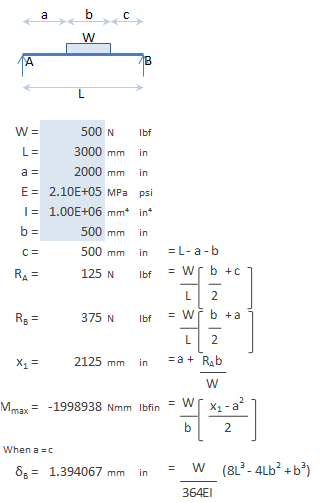Native Excel:
Click in the text area below and copy.
Paste into cell A1 of a worksheet.

XLC Enhanced Excel:
Use XLC’s ‘Web Paste’ button to paste at any position in worksheet.
Includes mathematical formatting and XLC equations.
Note: Both include SI(mm) & US(in) units (delete as appropriate).

### Case 2) Point Load on Simply Supported Beam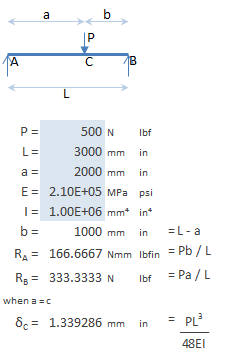Native Excel:
Click in the text area below and copy.
Paste into cell A1 of a worksheet.

XLC Enhanced Excel:
Use XLC’s ‘Web Paste’ button to paste at any position in worksheet.
Includes mathematical formatting and XLC equations.
Note: Both include SI(mm) & US(in) units (delete as appropriate).

### Case 3) Applied Moment on Simply Supported Beam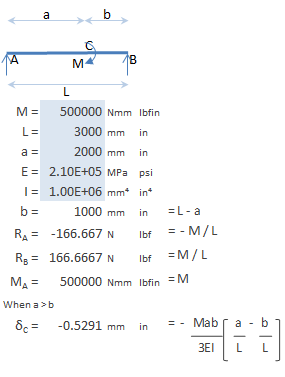Native Excel:
Click in the text area below and copy.
Paste into cell A1 of a worksheet.

XLC Enhanced Excel:
Use XLC’s ‘Web Paste’ button to paste at any position in worksheet.
Includes mathematical formatting and XLC equations.
Note: Both include SI(mm) & US(in) units (delete as appropriate).
 Purpose of calculation Calculate reaction forces, moment and deflection in cantilever beams.     Calculation Reference Steel Designer's Manual 5th Edition Calculation Validation All cell formulas checked with XLC equations against calculation reference. Copy HTML code snippet to create a dynamic window to this page from your own website Taken from the ExcelCalcs.com website.
Nomenclature Young's Modulus = E Beam 2nd moment of area = I Applied distributed load = W Applied point load = P Moment = M Reaction force = R   Deflection = δ

### Case 1) Uniformly Distributed Load on Cantilever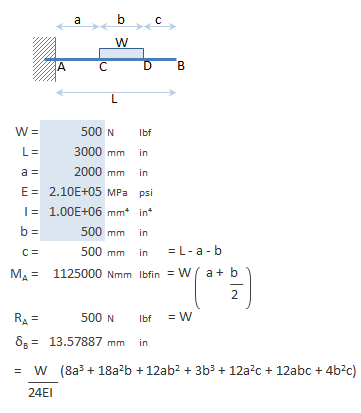Native Excel:
Click in the text area below and copy.
Paste into cell A1 of a worksheet.

XLC Enhanced Excel:
Use XLC’s ‘Web Paste’ button to paste at any position in worksheet.
Includes mathematical formatting and XLC equations.
Note: Both include SI(mm) & US(in) units (delete as appropriate).

### Case 2) Point Load on Cantilever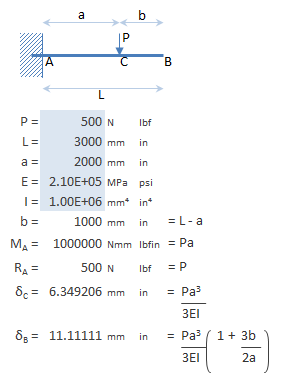Native Excel:
Click in the text area below and copy.
Paste into cell A1 of a worksheet.

XLC Enhanced Excel:
Use XLC’s ‘Web Paste’ button to paste at any position in worksheet.
Includes mathematical formatting and XLC equations.
Note: Both include SI(mm) & US(in) units (delete as appropriate).

### Case 3) Applied Moment on Cantilever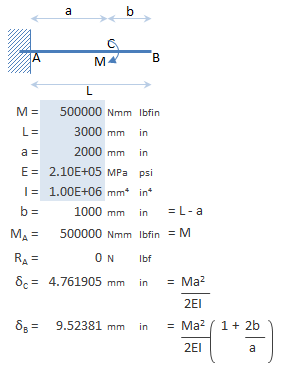Native Excel:
Click in the text area below and copy.
Paste into cell A1 of a worksheet.

XLC Enhanced Excel:
Use XLC’s ‘Web Paste’ button to paste at any position in worksheet.
Includes mathematical formatting and XLC equations.
Note: Both include SI(mm) & US(in) units (delete as appropriate).

### Case 1) Uniformly Distributed Load on Simply Supported Beam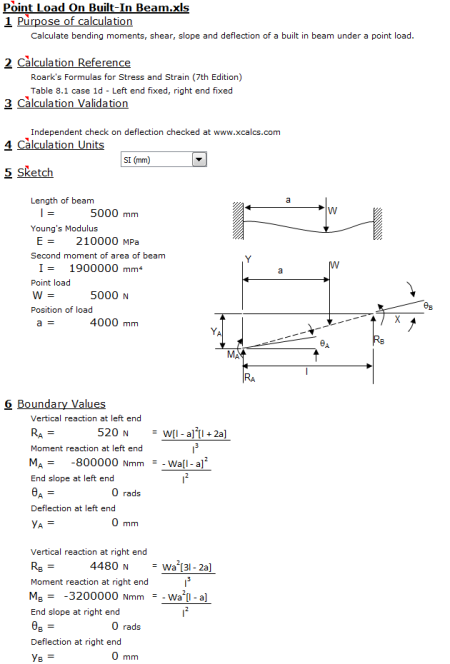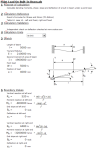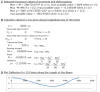Native Excel:
Click in the text area below and copy.
Paste into cell A1 of a worksheet.

XLC Enhanced Excel:
Use XLC’s ‘Web Paste’ button to paste at any position in worksheet.
Includes mathematical formatting and XLC equations.
Note: Both include SI(mm) & US(in) units (delete as appropriate).